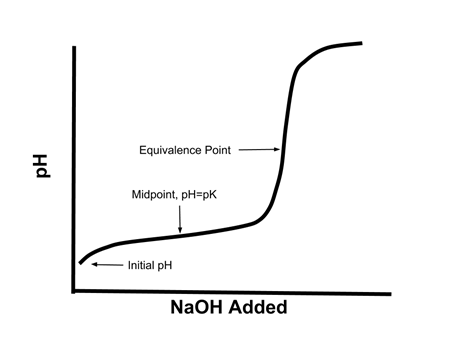# Enduring Understanding 6.C.2: Buffers

• Consider again the titration curve of a weak acid:
•• Notice how in the middle of the curve, especially around the midpoint, there is a relatively small change in pH when a given amount of strong base is added.
• This is an illustration of a property of buffers - systems where the addition of small amounts of acid or base do not greatly change the pH of the system

• A buffer contains significant concentrations of a conjugate acid-base pair. When strong acid or base is added, they react with either the weak acid or the weak base, and the pH does not change much.
• Weak acids and their conjugate bases, or weak bases and their conjugate acids make good buffers.
• Examples include acetic acid/acetate, pyridinium/pyridine, carbonic acid/hydrogen carbonate...
• Buffers are best able to resist changes in pH when there is a near equal concentration of the conjugate acid and base (i.e. when the pH = pKa of the acid/base pair).
• Example: To create a buffer with a pH of 5.5, which of the following acid/base pairs would we choose? HCOOH/HCOONa (pKa = 3.7); C5H5N/C5H5NHCl (pKa = 5.2); HClO/NaOCl (pKa = 7.5).
• Pyridine/pyridinium chloride would form the best pH = 5.5 buffer, because its pKa (5.2) is closest to 5.5.
• A buffer can only resist changes in pH as long as some of each of the acid and base remain. If they are consumed (for example, by the addition of a large amount of strong acid or base) the solution is no longer a buffer.

• Sample Question: A 1L buffer solution of 0.1M acetic acid and 0.1M sodium acetate will have its buffering capacity destroyed by the addition of which of the following?
• a) 0.2 mol sodium acetate
b) 0.3 mol acetic acid
c) 0.05 mol sodium hydroxide
d) 0.25 mol hydrochloric acid

• Answer: (d), 0.25 mol hydrochloric acid.
• As long as there is some acetic acid and sodium acetate present, the system is a buffer, so (a) and (b) will have no effect. (c), sodium hydroxide, will consume some of the acetic acid, but there is not enough to consume all the acetic acid (0.1 mol) present. However, 0.25 mol of HCl is enough to consume all the sodium acetate (0.1 mol) present, so this will remove the buffering capacity of the solution.

 Related Links: Chemistry Chemistry Quizzes AP Chemistry Notes Acid-Base Reactions AP Chemistry Quizzes Atoms, Molecules, and Chemical Analysis Quiz Electronic Structure of Atoms Quiz Periodicity, Atomic & Ionic Radii, Atomic Models Quiz Conservation of Matter and Balanced Equations Quiz Gases and Solutions Quiz Intermolecular Forces Quiz Molecular Geometry and VSEPR Quiz Bonding and Solid State Properties Quiz Balanced and Ionic Equations Quiz Chemical Reactions Quiz Redox Reactions and Electrochemistry Quiz Chemical Kinetics and Rates Quiz Reaction Rates and Collisions Quiz Multi-Step Reaction Rates and Catalysis Quiz Energy and Heat Quiz Conservation of Energy and Calorimetry Quiz Bond Energies Quiz Entropy and Gibbs Free Energy Quiz Chemical Equilibrium Quiz Solubility and Acid-Base Equilibrium Quiz

To link to this Buffers page, copy the following code to your site: